# Bridges SDE’s

Consider now a $$d$$-dimensional stochastic process $$X_{t}$$ defined on a probability space $$(\Omega, \mathfrak{F},\mathbb{P})$$. We say that the bridge associated to $$X_{t}$$ conditioned to the event $$\{X_{T}= a\}$$ is the process: $\{\tilde{X}_{t}, t_{0} \leq t \leq T \}=\{X_{t}, t_{0} \leq t \leq T: X_{T}= a \}$ where $$T$$ is a deterministic fixed time and $$a \in \mathbb{R}^d$$ is fixed too.

# The bridgesdekd() functions

The (S3) generic function bridgesdekd() (where k=1,2,3) for simulation of 1,2 and 3-dim bridge stochastic differential equations,It? or Stratonovich type, with different methods. The main arguments consist:

• The drift and diffusion coefficients as R expressions that depend on the state variable x (y and z) and time variable t.
• The number of simulation steps N.
• The number of the solution trajectories to be simulated by M (default: M=1).
• Initial value x0 at initial time t0.
• Terminal value y final time T
• The integration step size Dt (default: Dt=(T-t0)/N).
• The choice of process types by the argument type="ito" for Ito or type="str" for Stratonovich (by default type="ito").
• The numerical method to be used by method (default method="euler").

By Monte-Carlo simulations, the following statistical measures (S3 method) for class bridgesdekd() (where k=1,2,3) can be approximated for the process at any time $$t \in [t_{0},T]$$ (default: at=(T-t0)/2):

• The expected value $$\text{E}(X_{t})$$ at time $$t$$, using the command mean.
• The variance $$\text{Var}(X_{t})$$ at time $$t$$, using the command moment with order=2 and center=TRUE.
• The median $$\text{Med}(X_{t})$$ at time $$t$$, using the command Median.
• The mode $$\text{Mod}(X_{t})$$ at time $$t$$, using the command Mode.
• The quartile of $$X_{t}$$ at time $$t$$, using the command quantile.
• The maximum and minimum of $$X_{t}$$ at time $$t$$, using the command min and max.
• The skewness and the kurtosis of $$X_{t}$$ at time $$t$$, using the command skewness and kurtosis.
• The coefficient of variation (relative variability) of $$X_{t}$$ at time $$t$$, using the command cv.
• The central moments up to order $$p$$ of $$X_{t}$$ at time $$t$$, using the command moment.
• The result summaries of the results of Monte-Carlo simulation at time $$t$$, using the command summary.

We can just make use of the rsdekd() function (where k=1,2,3) to build our random number for class bridgesdekd() (where k=1,2,3) at any time $$t \in [t_{0},T]$$. the main arguments consist:

• object an object inheriting from class bridgesdekd() (where k=1,2,3).
• at time between $$s=t0$$ and $$t=T$$.

The function dsde() (where k=1,2,3) approximate transition density for class bridgesdekd() (where k=1,2,3), the main arguments consist:

• object an object inheriting from class bridgesdekd() (where k=1,2,3).
• at time between $$s=t0$$ and $$t=T$$.
• pdf probability density function Joint or Marginal.

The following we explain how to use this functions.

# bridgesde1d()

Assume that we want to describe the following bridge sde in It? form: $\begin{equation}\label{eq0166} dX_t = \frac{1-X_t}{1-t} dt + X_t dW_{t},\quad X_{t_{0}}=3 \quad\text{and}\quad X_{T}=1 \end{equation}$ We simulate a flow of $$1000$$ trajectories, with integration step size $$\Delta t = 0.001$$, and $$x_0 = 3$$ at time $$t_0 = 0$$, $$y = 1$$ at terminal time $$T=1$$.

R> f <- expression((1-x)/(1-t))
R> g <- expression(x)
R> mod <- bridgesde1d(drift=f,diffusion=g,x0=3,y=1,M=1000,method="milstein")
R> mod
Itô Bridge Sde 1D:
| dX(t) = (1 - X(t))/(1 - t) * dt + X(t) * dW(t)
Method:
| First-order Milstein scheme
Summary:
| Size of process   | N = 1001.
| Crossing realized | C = 979 among 1000.
| Initial value     | x0 = 3.
| Ending value      | y = 1.
| Time of process   | t in [0,1].
| Discretization    | Dt = 0.001.
R> summary(mod) ## default: summary at time = (T-t0)/2

Monte-Carlo Statistics for X(t) at time t = 0.5
| Crossing realized 979 among 1000

Mean               1.94186
Variance           1.43874
Median             1.60383
Mode               1.21092
First quartile     1.10418
Third quartile     2.39639
Minimum            0.39455
Maximum            9.53637
Skewness           1.81039
Kurtosis           7.64157
Coef-variation     0.61769
3th-order moment   3.12424
4th-order moment  15.81775
5th-order moment  78.18722
6th-order moment 450.77324

In Figure 1, we present the flow of trajectories, the mean path (red lines) of solution of $$X_{t}|X_{0}=3,X_{T}=1$$:

R> plot(mod,ylab=expression(X[t]))
R> lines(time(mod),apply(mod$X,1,mean),col=2,lwd=2) R> legend("topleft","mean path",inset = .01,col=2,lwd=2,cex=0.8,bty="n")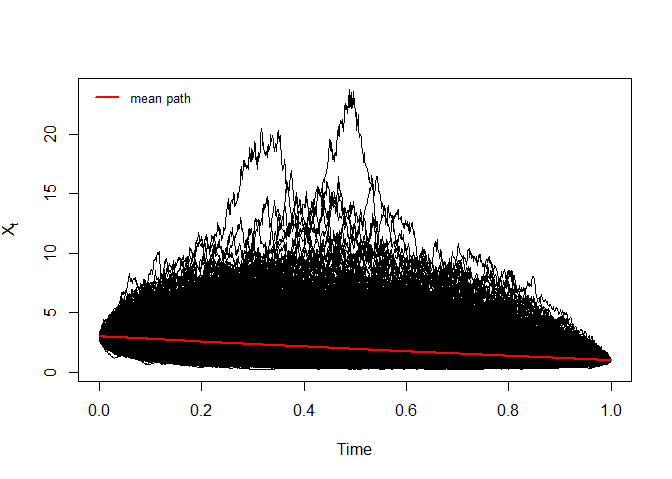Bridge sde 1D Figure 2, show approximation results for $$m(t)=\text{E}(X_{t}|X_{0}=3,X_{T}=1)$$ and $$S(t)=\text{V}(X_{t}|X_{0}=3,X_{T}=1)$$: R> m <- apply(mod$X,1,mean)
R> S  <- apply(mod$X,1,var) R> out <- data.frame(m,S) R> matplot(time(mod), out, type = "l", xlab = "time", ylab = "", col=2:3,lwd=2,lty=2:3,las=1) R> legend("topright",c(expression(m(t),S(t))),col=2:3,lty=2:3,lwd=2,bty="n")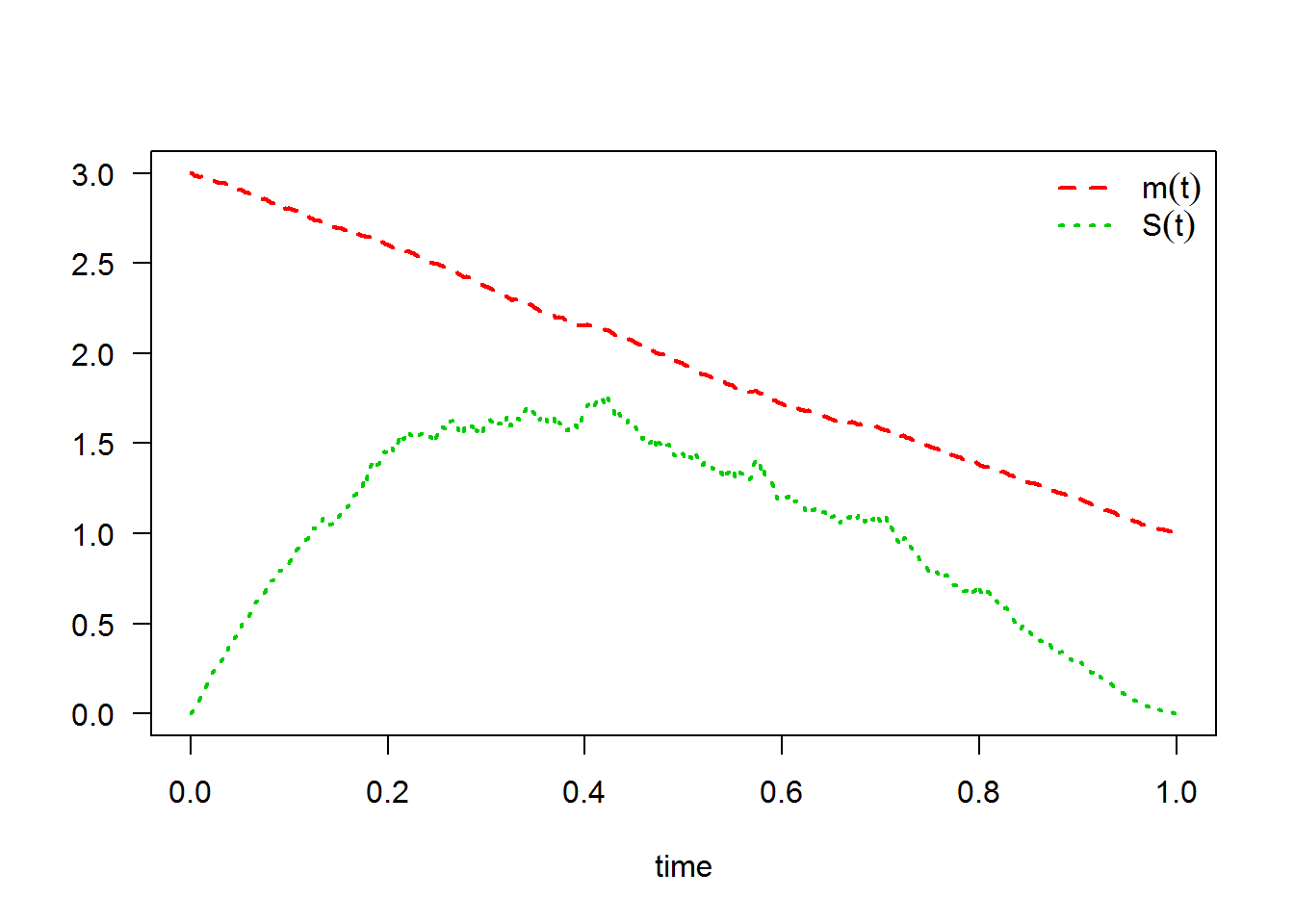The following statistical measures (S3 method) for class bridgesde1d() can be approximated for the $$X_{t}|X_{0}=3,X_{T}=1$$ process at any time $$t$$, for example at=0.55: R> s = 0.55 R> mean(mod, at = s)  1.8205 R> moment(mod, at = s , center = TRUE , order = 2) ## variance  1.352 R> Median(mod, at = s)  1.5021 R> Mode(mod, at = s)  1.1577 R> quantile(mod , at = s)  0% 25% 50% 75% 100% 0.27357 1.05000 1.50215 2.19822 9.97644  R> kurtosis(mod , at = s)  9.052 R> skewness(mod , at = s)  2.0658 R> cv(mod , at = s )  0.63904 R> min(mod , at = s)  0.27357 R> max(mod , at = s)  9.9764 R> moment(mod, at = s , center= TRUE , order = 4)  16.581 R> moment(mod, at = s , center= FALSE , order = 4)  78.134 The result summaries of the $$X_{t}|X_{0}=3,X_{T}=1$$ process at time $$t=0.55$$: R> summary(mod, at = 0.55)  Monte-Carlo Statistics for X(t) at time t = 0.55 | Crossing realized 979 among 1000 Mean 1.82047 Variance 1.35340 Median 1.50215 Mode 1.15773 First quartile 1.05000 Third quartile 2.19822 Minimum 0.27357 Maximum 9.97644 Skewness 2.06585 Kurtosis 9.05203 Coef-variation 0.63904 3th-order moment 3.25266 4th-order moment 16.58061 5th-order moment 87.02682 6th-order moment 534.81157 Hence we can just make use of the rsde1d() function to build our random number generator for $$X_{t}|X_{0}=3,X_{T}=1$$ at time $$t=0.55$$: R> x <- rsde1d(object = mod, at = s) R> head(x, n = 10)   1.30209 1.66625 4.00433 2.39197 0.94416 4.04228 1.15678 2.70037  1.35054 0.57208 R> summary(x)  Min. 1st Qu. Median Mean 3rd Qu. Max. 0.274 1.050 1.502 1.821 2.198 9.976  Display the random number generator for $$X_{t}|X_{0}=3,X_{T}=1$$, see Figure 3: R> plot(time(mod),mod$X[,1],type="l",ylab="X(t)",xlab="time",axes=F,lty=3)
R> points(s,x,pch=19,col=2,cex=0.5)
R> lines(c(s,s),c(0,x),lty=2,col=2)
R> lines(c(0,s),c(x,x),lty=2,col=2)
R> axis(1, s, bquote(at==.(s)),col=2,col.ticks=2)
R> axis(2, x, bquote(X[t==.(s)]),col=2,col.ticks=2)
R> legend('topright',col=2,pch=19,legend=bquote(X[t==.(s)]==.(x)),bty = 'n')
R> box()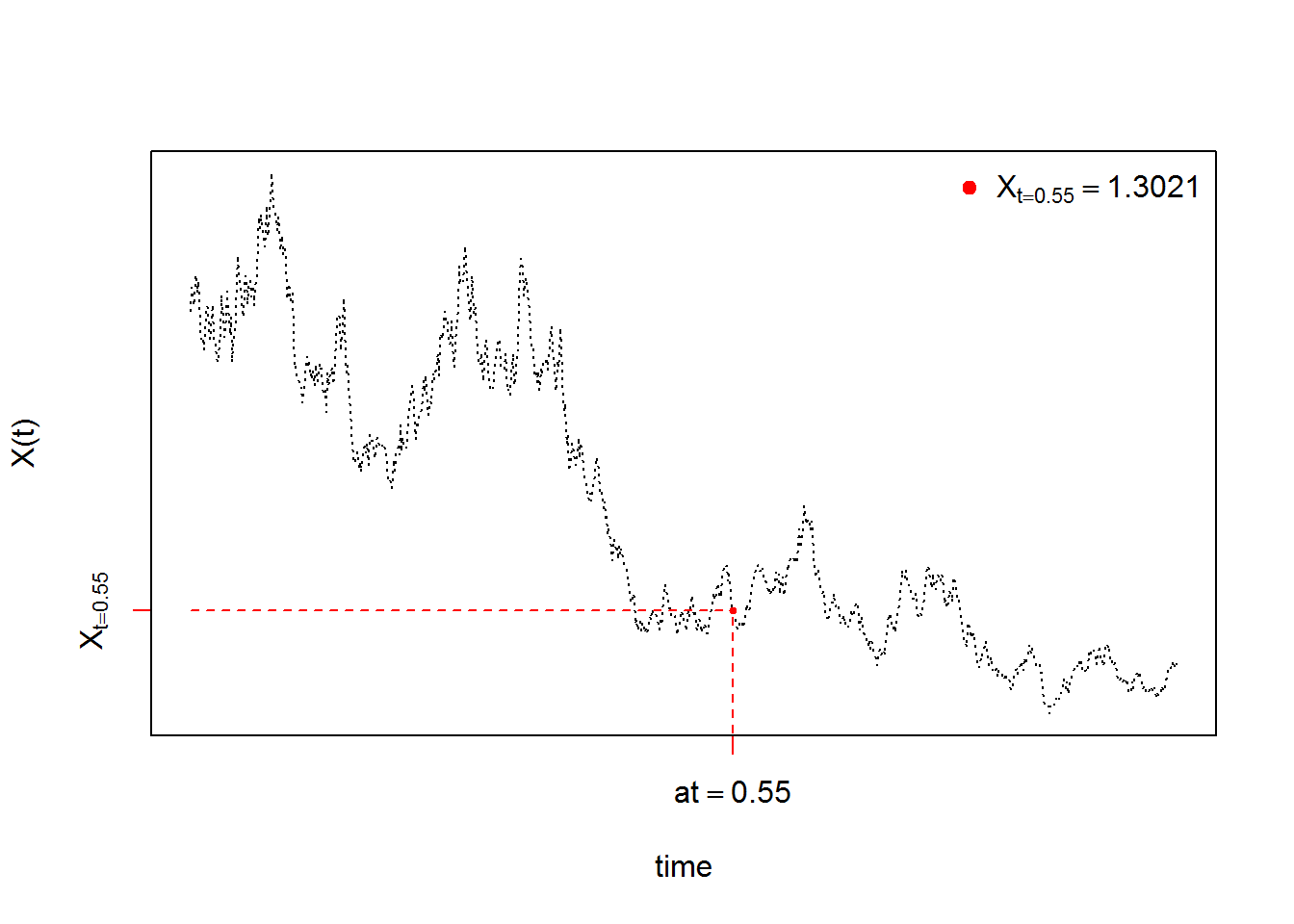The function dsde1d() can be used to show the kernel density estimation for $$X_{t}|X_{0}=3,X_{T}=1$$ at time $$t=0.55$$ (hist=TRUE based on truehist() function in MASS package):

R> dens <- dsde1d(mod, at = s)
R> dens

Density of X(t-t0)|X(t0) = 3, X(T) = 1 at time t = 0.55

Data: x (979 obs.); Bandwidth 'bw' = 0.1945

x                f(x)
Min.   :-0.3101   Min.   :0.00000
1st Qu.: 2.4075   1st Qu.:0.00115
Median : 5.1250   Median :0.01298
Mean   : 5.1250   Mean   :0.09191
3rd Qu.: 7.8425   3rd Qu.:0.10136
Max.   :10.5601   Max.   :0.56099  
R> plot(dens,hist=TRUE) ## histgramme
R> plot(dens,add=TRUE)  ## kernel density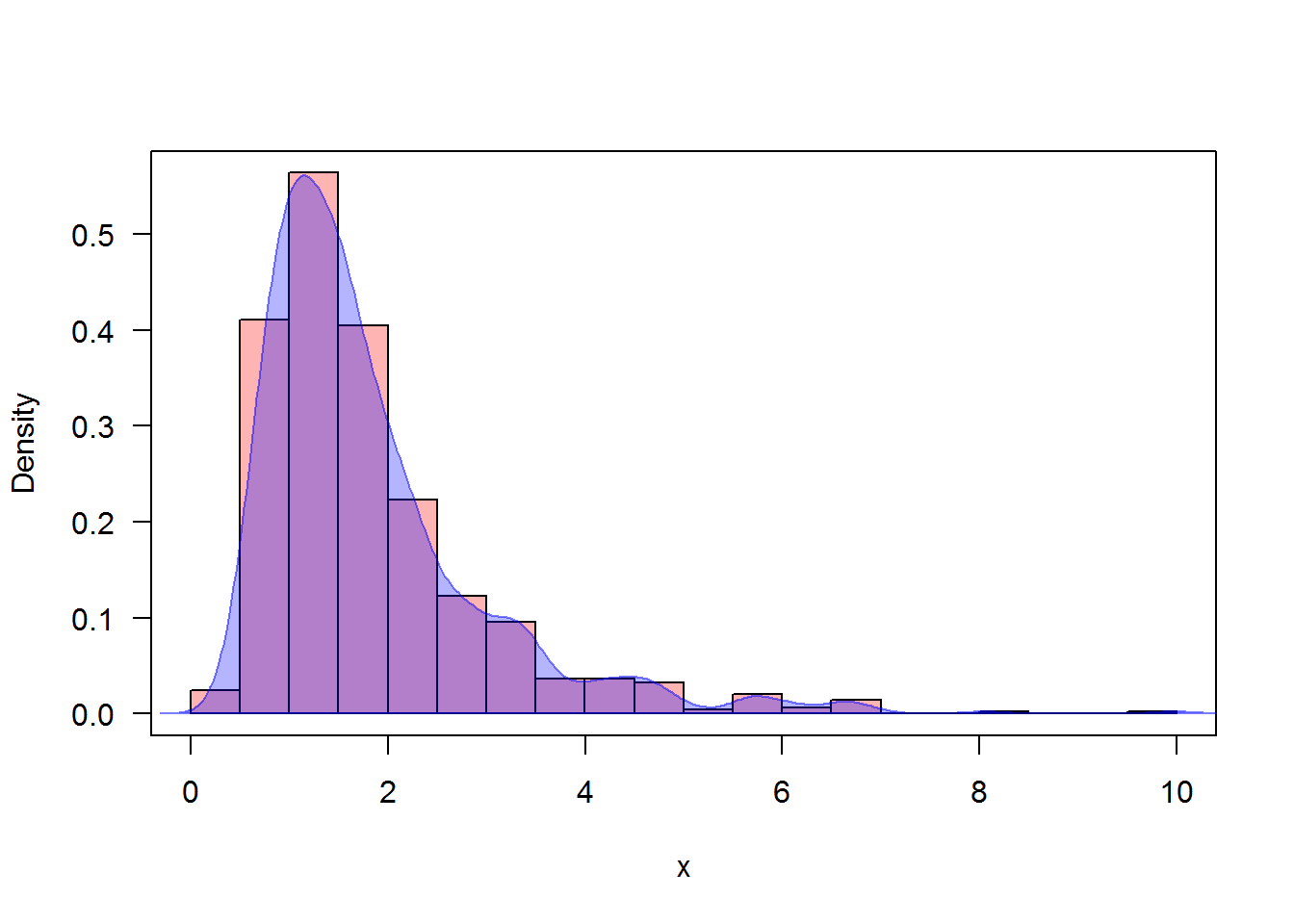Approximate the transitional densitie of $$X_{t}|X_{0}=3,X_{T}=1$$ at $$t-s = \{0.25,0.75\}$$:

R> plot(dsde1d(mod,at=0.75))
R> legend('topright',col=c('#0000FF4B','#FF00004B'),pch=15,legend=c("t-s=0.25","t-s=0.75"),bty = 'n')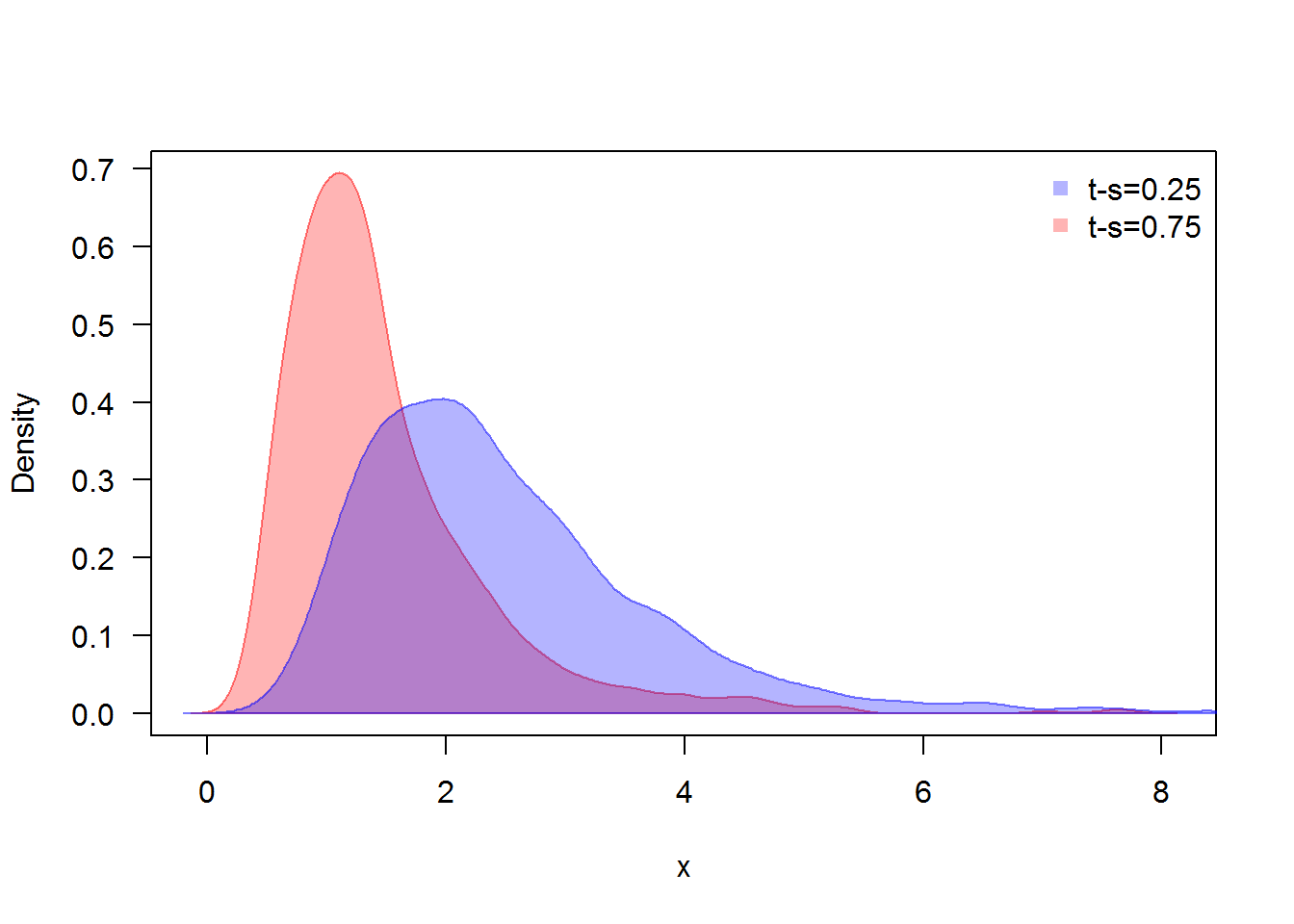Transitional densitie at time $$t-s = 0.25,0.75$$

# bridgesde2d()

Assume that we want to describe the following $$2$$-dimensional bridge SDE’s in Stratonovich form:

$\begin{equation}\label{eq:09} \begin{cases} dX_t = -(1+Y_{t}) X_{t} dt + 0.2 (1-Y_{t})\circ dW_{1,t},\quad X_{t_{0}}=1 \quad\text{and}\quad X_{T}=1\\ dY_t = -(1+X_{t}) Y_{t} dt + 0.1 (1-X_{t}) \circ dW_{2,t},\quad Y_{t_{0}}=-0.5 \quad\text{and}\quad Y_{T}=0.5 \end{cases} \end{equation}$

We simulate a flow of $$1000$$ trajectories, with integration step size $$\Delta t = 0.01$$, and using Runge-Kutta method order 1:

R> fx <- expression(-(1+y)*x , -(1+x)*y)
R> gx <- expression(0.2*(1-y),0.1*(1-x))
R> mod2 <- bridgesde2d(drift=fx,diffusion=gx,x0=c(1,-0.5),y=c(1,0.5),Dt=0.01,M=1000,type="str",method="rk1")
R> mod2
Stratonovich Bridge Sde 2D:
| dX(t) = -(1 + Y(t)) * X(t) * dt + 0.2 * (1 - Y(t)) o dW1(t)
| dY(t) = -(1 + X(t)) * Y(t) * dt + 0.1 * (1 - X(t)) o dW2(t)
Method:
| Runge-Kutta method with order 1
Summary:
| Size of process   | N = 1001.
| Crossing realized | C = 999 among 1000.
| Initial values    | x0 = (1,-0.5).
| Ending values     | y = (1,0.5).
| Time of process   | t in [0,10].
| Discretization    | Dt = 0.01.
R> summary(mod2) ## default: summary at time = (T-t0)/2

Monte-Carlo Statistics for (X(t),Y(t)) at time t = 5
| Crossing realized 999 among 1000
X          Y
Mean              0.01893    0.00003
Variance          0.01934    0.00550
Median            0.01989   -0.00121
Mode             -0.01072    0.00390
First quartile   -0.07727   -0.04825
Third quartile    0.11293    0.04953
Minimum          -0.49455   -0.21025
Maximum           0.48453    0.28688
Skewness         -0.00191    0.15973
Kurtosis          3.11148    3.37163
Coef-variation    7.34857 2676.28321
3th-order moment -0.00001    0.00007
4th-order moment  0.00116    0.00010
5th-order moment -0.00001    0.00001
6th-order moment  0.00012    0.00000

In Figure 6, we present the flow of trajectories of $$X_{t}|X_{0}=1,X_{T}=1$$ and $$Y_{t}|Y_{0}=-0.5,Y_{T}=0.5$$:

R> plot(mod2,col=c('#FF00004B','#0000FF82'))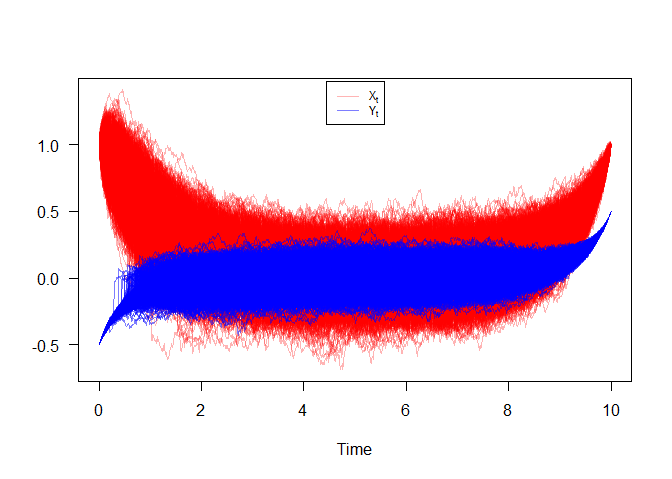Bridge sde 2D

Figure 7, show approximation results for $$m_{1}(t)=\text{E}(X_{t}|X_{0}=1,X_{T}=1)$$, $$m_{2}(t)=\text{E}(Y_{t}|Y_{0}=-0.5,Y_{T}=0.5)$$,and $$S_{1}(t)=\text{V}(X_{t}|X_{0}=1,X_{T}=1)$$, $$S_{2}(t)=\text{V}(Y_{t}|Y_{0}=-0.5,Y_{T}=0.5)$$, and $$C_{12}(t)=\text{COV}(X_{t},Y_{t}|X_{0}=1,Y_{0}=-0.5,X_{T}=1,Y_{T}=0.5)$$:

R> m1  <- apply(mod2$X,1,mean) R> m2 <- apply(mod2$Y,1,mean)
R> S1  <- apply(mod2$X,1,var) R> S2 <- apply(mod2$Y,1,var)
R> C12 <- sapply(1:dim(mod2$X),function(i) cov(mod2$X[i,],mod2$Y[i,])) R> out2 <- data.frame(m1,m2,S1,S2,C12) R> matplot(time(mod2), out2, type = "l", xlab = "time", ylab = "", col=2:6,lwd=2,lty=2:6,las=1) R> legend("top",c(expression(m(t),m(t),S(t),S(t),C(t))),col=2:6,lty=2:6,lwd=2,bty="n")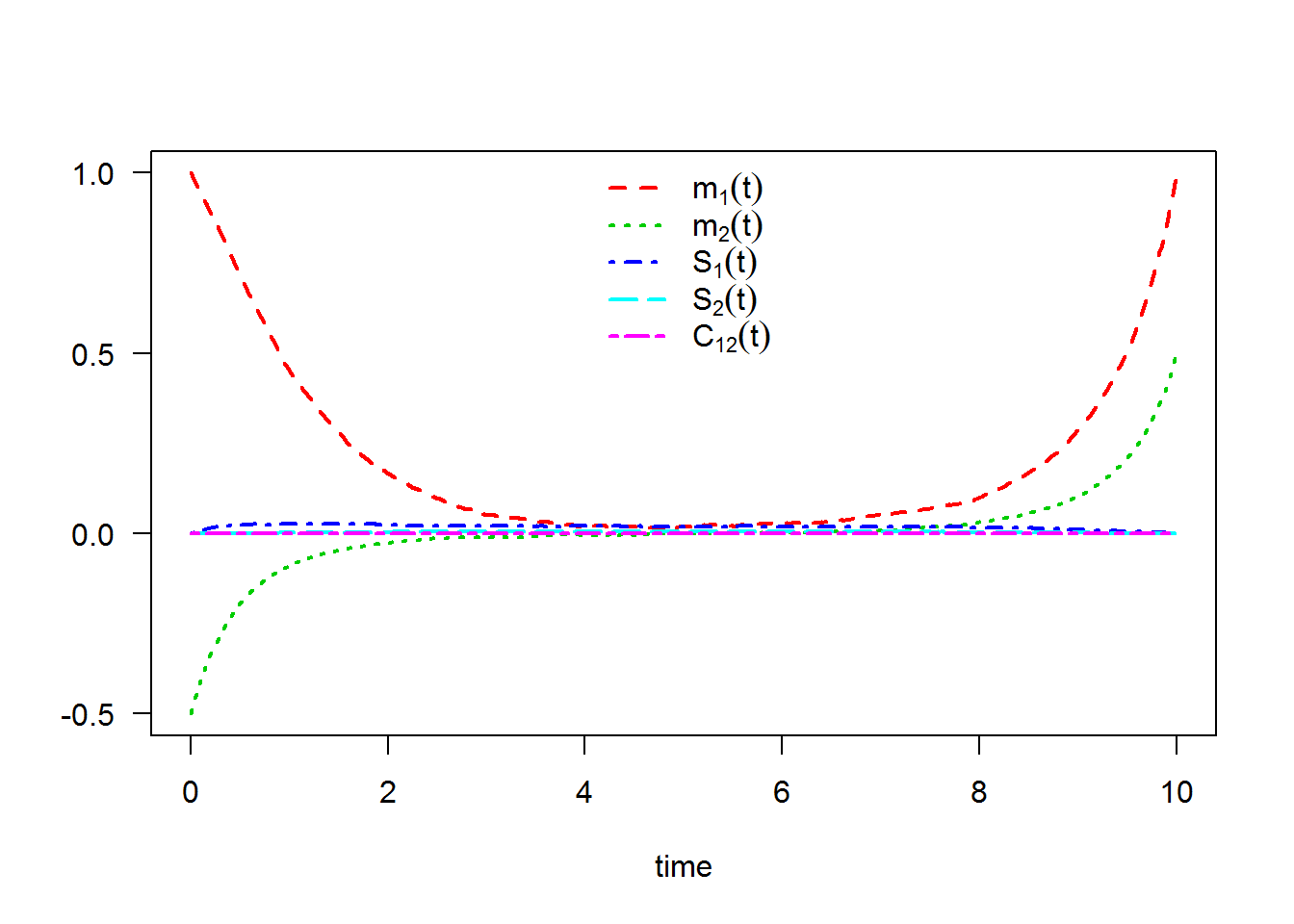The following statistical measures (S3 method) for class bridgesde2d() can be approximated for the $$X_{t}|X_{0}=1,X_{T}=1$$ and $$Y_{t}|Y_{0}=-0.5,Y_{T}=0.5$$ process at any time $$t$$, for example at=6.75: R> s = 6.75 R> mean(mod2, at = s)  0.0429152 0.0063793 R> moment(mod2, at = s , center = TRUE , order = 2) ## variance  0.0194081 0.0045834 R> Median(mod2, at = s)  0.0450432 0.0067505 R> Mode(mod2, at = s)  0.0728426 0.0023237 R> quantile(mod2 , at = s) $x
0%       25%       50%       75%      100%
-0.430771 -0.049729  0.045043  0.131096  0.464601

$y 0% 25% 50% 75% 100% -0.2807212 -0.0368485 0.0067505 0.0528729 0.2477952  R> kurtosis(mod2 , at = s)  3.2022 3.6669 R> skewness(mod2 , at = s)  -0.0056966 -0.1017900 R> cv(mod2 , at = s )  3.2479 10.6178 R> min(mod2 , at = s)  -0.43077 -0.28072 R> max(mod2 , at = s)  0.4646 0.2478 R> moment(mod2 , at = s , center= TRUE , order = 4)  0.001208612 0.000077186 R> moment(mod2 , at = s , center= FALSE , order = 4)  0.0014238 0.0000775 The result summaries of the $$X_{t}|X_{0}=1,X_{T}=1$$ and $$Y_{t}|Y_{0}=-0.5,Y_{T}=0.5$$ process at time $$t=6.75$$: R> summary(mod2, at = 6.75)  Monte-Carlo Statistics for (X(t),Y(t)) at time t = 6.75 | Crossing realized 999 among 1000 X Y Mean 0.04292 0.00638 Variance 0.01943 0.00459 Median 0.04504 0.00675 Mode 0.07284 0.00232 First quartile -0.04973 -0.03685 Third quartile 0.13110 0.05287 Minimum -0.43077 -0.28072 Maximum 0.46460 0.24780 Skewness -0.00570 -0.10179 Kurtosis 3.20222 3.66689 Coef-variation 3.24786 10.61779 3th-order moment -0.00002 -0.00003 4th-order moment 0.00121 0.00008 5th-order moment -0.00001 0.00000 6th-order moment 0.00012 0.00000 Hence we can just make use of the rsde2d() function to build our random number generator for the couple $$X_{t},Y_{t}|X_{0}=1,Y_{0}=-0.5,X_{T}=1,Y_{T}=0.5$$ at time $$t=6.75$$: R> x2 <- rsde2d(object = mod2, at = s) R> head(x2, n = 10)  x y 1 0.0802863 -0.0592030 2 -0.0593623 0.0563347 3 -0.0093671 -0.0492190 4 0.1135604 -0.0031016 5 0.0270850 -0.0275360 6 -0.0756099 0.0637535 7 -0.0940426 0.0792022 8 -0.3467712 0.0367189 9 -0.0426078 -0.0507953 10 0.2329908 0.0171084 R> summary(x2)  x y Min. :-0.4308 Min. :-0.28072 1st Qu.:-0.0497 1st Qu.:-0.03685 Median : 0.0450 Median : 0.00675 Mean : 0.0429 Mean : 0.00638 3rd Qu.: 0.1311 3rd Qu.: 0.05287 Max. : 0.4646 Max. : 0.24779  Display the random number generator for the couple $$X_{t},Y_{t}|X_{0}=1,Y_{0}=-0.5,X_{T}=1,Y_{T}=0.5$$, see Figure 8: R> plot(ts.union(mod2$X[,1],mod2$Y[,1]),col=1:2,lty=3,plot.type="single",type="l",ylab= "",xlab="time",axes=F) R> points(s,x2$x,pch=19,col=3,cex=0.8)
R> points(s,x2$y,pch=19,col=4,cex=0.8) R> lines(c(s,s),c(-10,x2$x),lty=2,col=6)
R> lines(c(0,s),c(x2$x,x2$x),lty=2,col=3)
R> lines(c(0,s),c(x2$y,x2$y),lty=2,col=4)
R> axis(1, s, bquote(at==.(s)),col=6,col.ticks=6)
R> axis(2, x2$x, bquote(X[t==.(s)]),col=3,col.ticks=3) R> axis(2, x2$y, bquote(Y[t==.(s)]),col=4,col.ticks=4)
R> legend('topright',legend=bquote(c(X[t==.(s)]==.(x2$x),Y[t==.(s)]==.(x2$y))),bty = 'n')
R> box()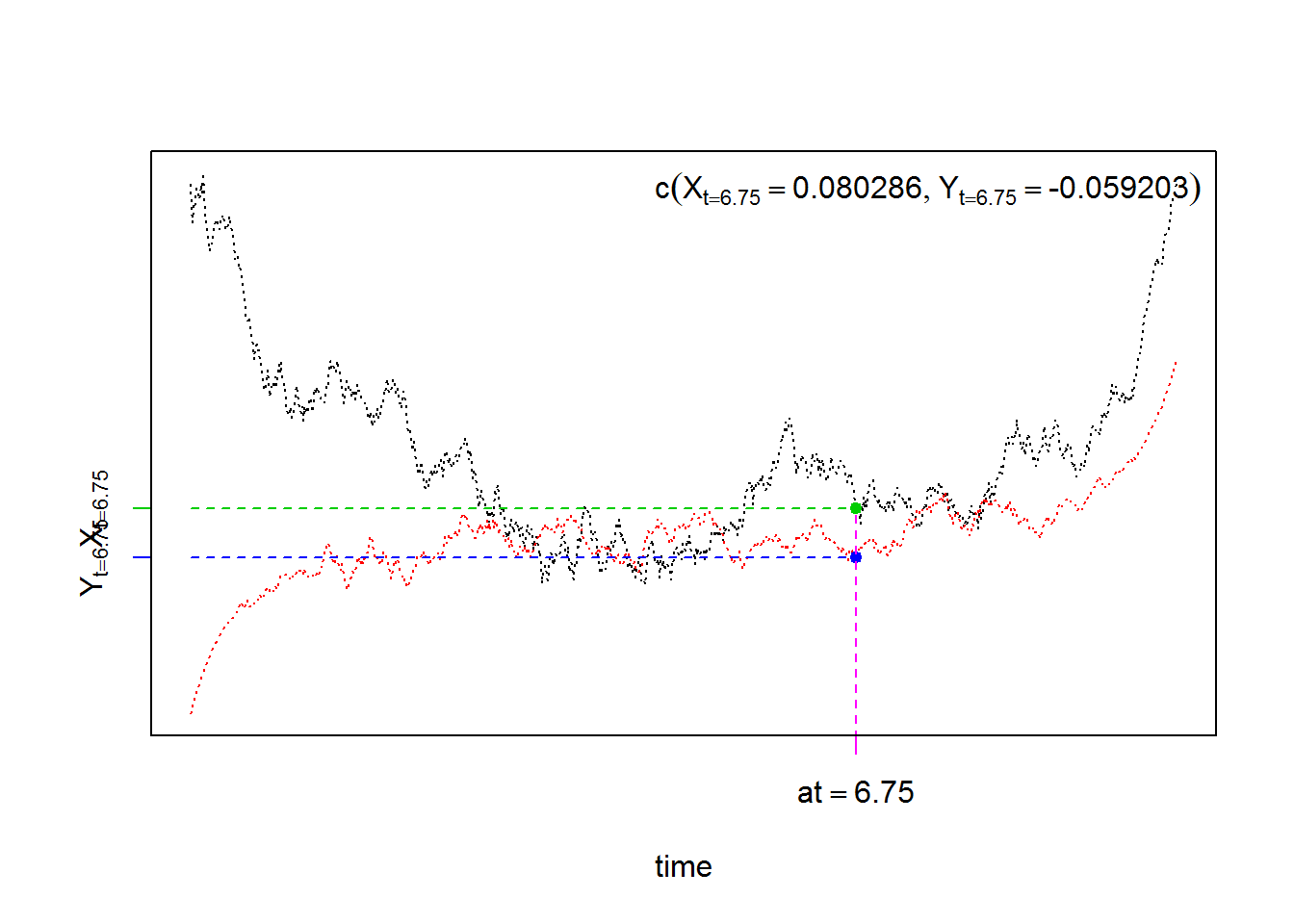For each SDE type and for each numerical scheme, the density of $$X_{t}|X_{0}=1,X_{T}=1$$ and $$Y_{t}|Y_{0}=-0.5,Y_{T}=0.5$$ at time $$t=6.75$$ are reported using dsde2d() function, see e.g. Figure 9:

R> denM <- dsde2d(mod2,pdf="M",at =s)
R> denM

Marginal density of X(t-t0)|X(t0) = 1, X(T) = 1 at time t = 6.75

Data: x (999 obs.); Bandwidth 'bw' = 0.03051

x                 f(x)
Min.   :-0.52231   Min.   :0.00016
1st Qu.:-0.25270   1st Qu.:0.06986
Median : 0.01692   Median :0.44116
Mean   : 0.01692   Mean   :0.92635
3rd Qu.: 0.28653   3rd Qu.:1.75750
Max.   : 0.55614   Max.   :2.79926

Marginal density of Y(t-t0)|Y(t0) = -0.5, Y(T) = 0.5 at time t = 6.75

Data: y (999 obs.); Bandwidth 'bw' = 0.01514

y                 f(y)
Min.   :-0.32614   Min.   :0.0003
1st Qu.:-0.17130   1st Qu.:0.0385
Median :-0.01646   Median :0.4104
Mean   :-0.01646   Mean   :1.6130
3rd Qu.: 0.13838   3rd Qu.:2.9482
Max.   : 0.29321   Max.   :5.9182  
R> plot(denM, main="Marginal Density")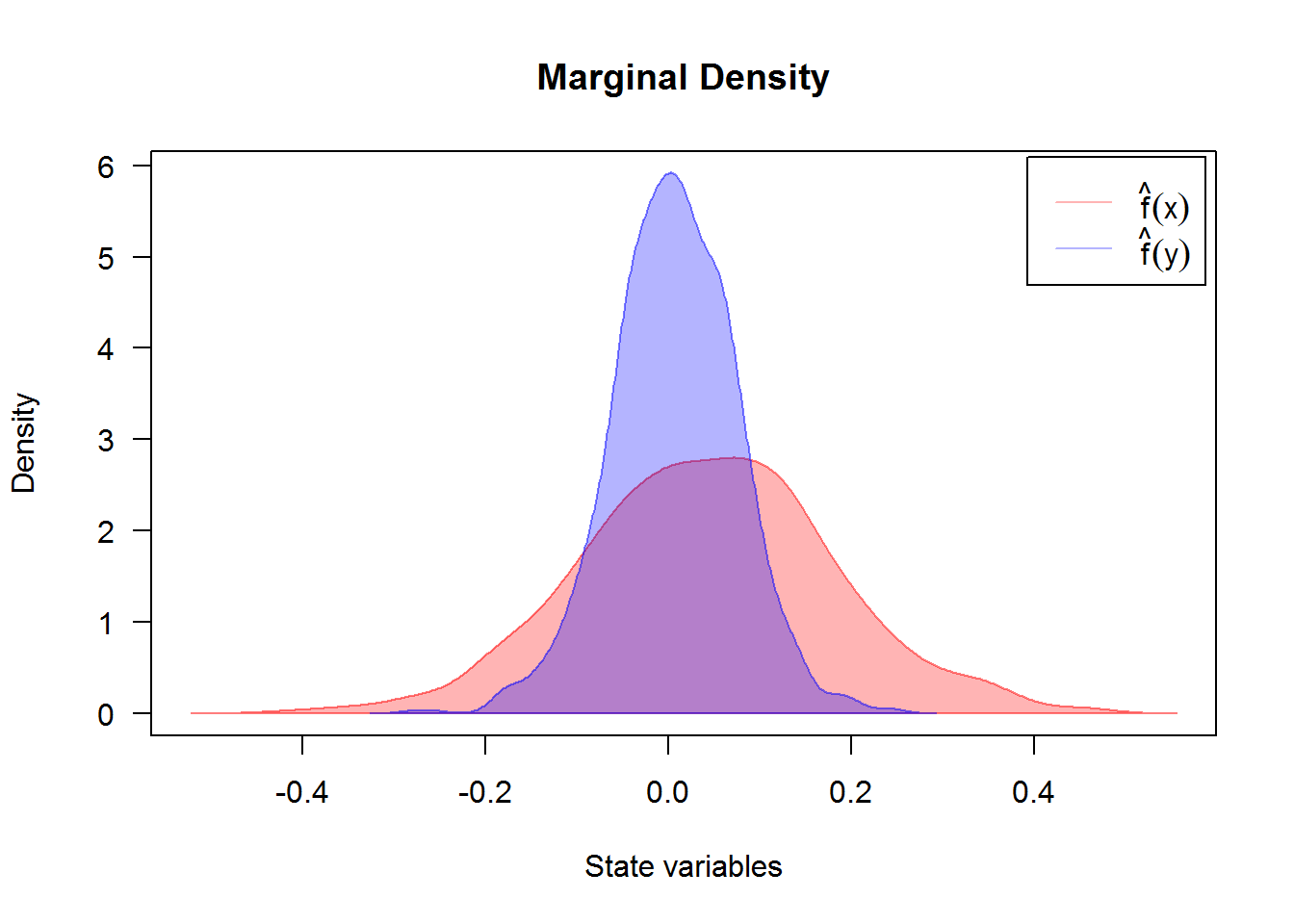Created using dsde2d() plotted in (x, y)-space with dim = 2. A contour and image plot of density obtained from a realization of the couple $$X_{t},Y_{t}|X_{0}=1,Y_{0}=-0.5,X_{T}=1,Y_{T}=0.5$$ at time t=6.75.

R> denJ <- dsde2d(mod2, pdf="J", n=100,at =s)
R> denJ

Joint density of (X(t-t0),Y(t-t0)|X(t0)=1,Y(t0)=-0.5,X(T)=1,Y(T)=0.5) at time t = 6.75

Data: (x,y) (2 x 999 obs.);

x                  y                 f(x,y)
Min.   :-0.43077   Min.   :-0.280721   Min.   : 0.0000
1st Qu.:-0.20693   1st Qu.:-0.148592   1st Qu.: 0.0406
Median : 0.01692   Median :-0.016463   Median : 0.3916
Mean   : 0.01692   Mean   :-0.016463   Mean   : 2.0637
3rd Qu.: 0.24076   3rd Qu.: 0.115666   3rd Qu.: 2.0635
Max.   : 0.46460   Max.   : 0.247795   Max.   :17.5065  
R> plot(denJ,display="contour",main="Bivariate Transition Density at time t=6.755")
R> plot(denJ,display="image",main="Bivariate Transition Density at time t=6.755")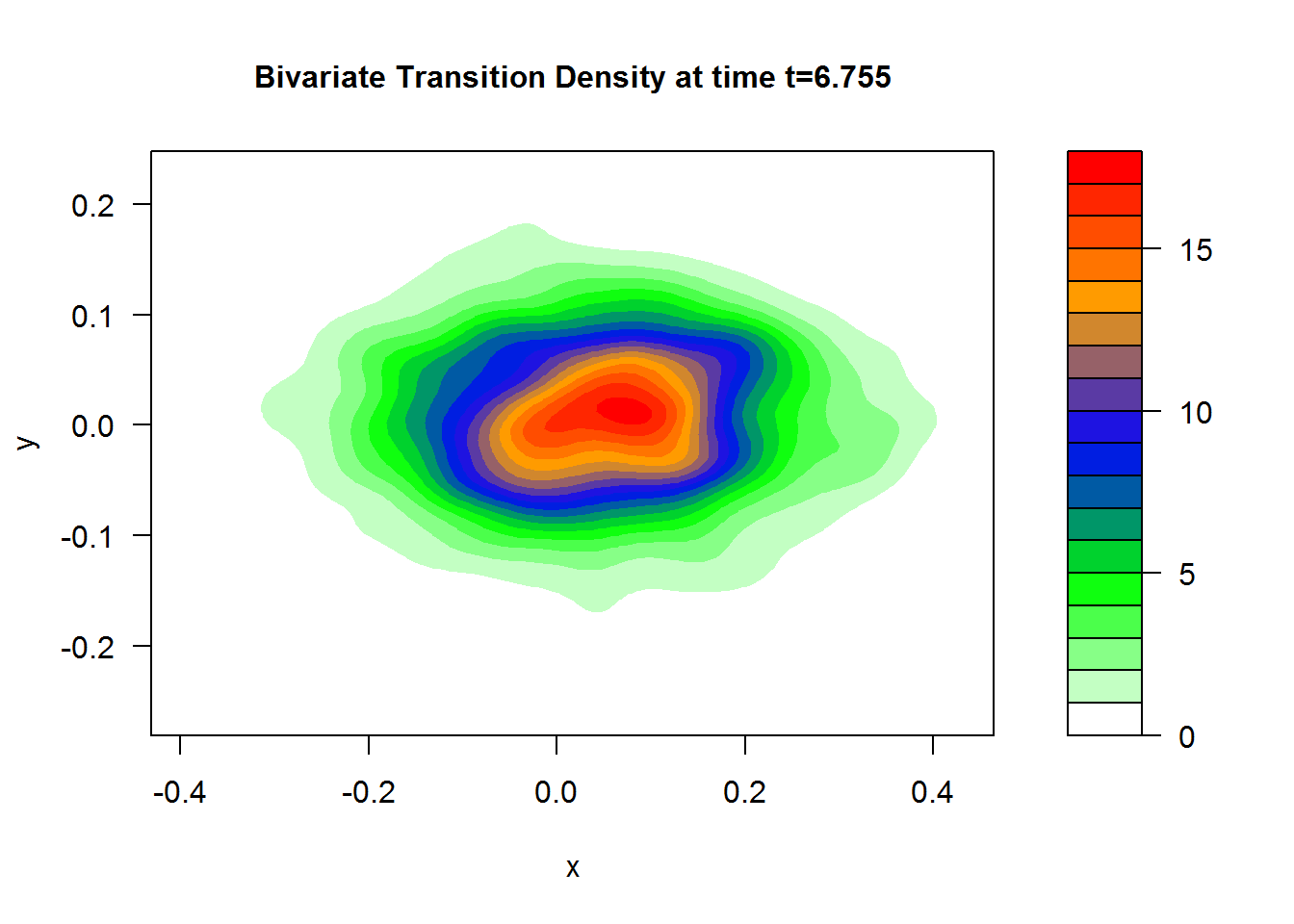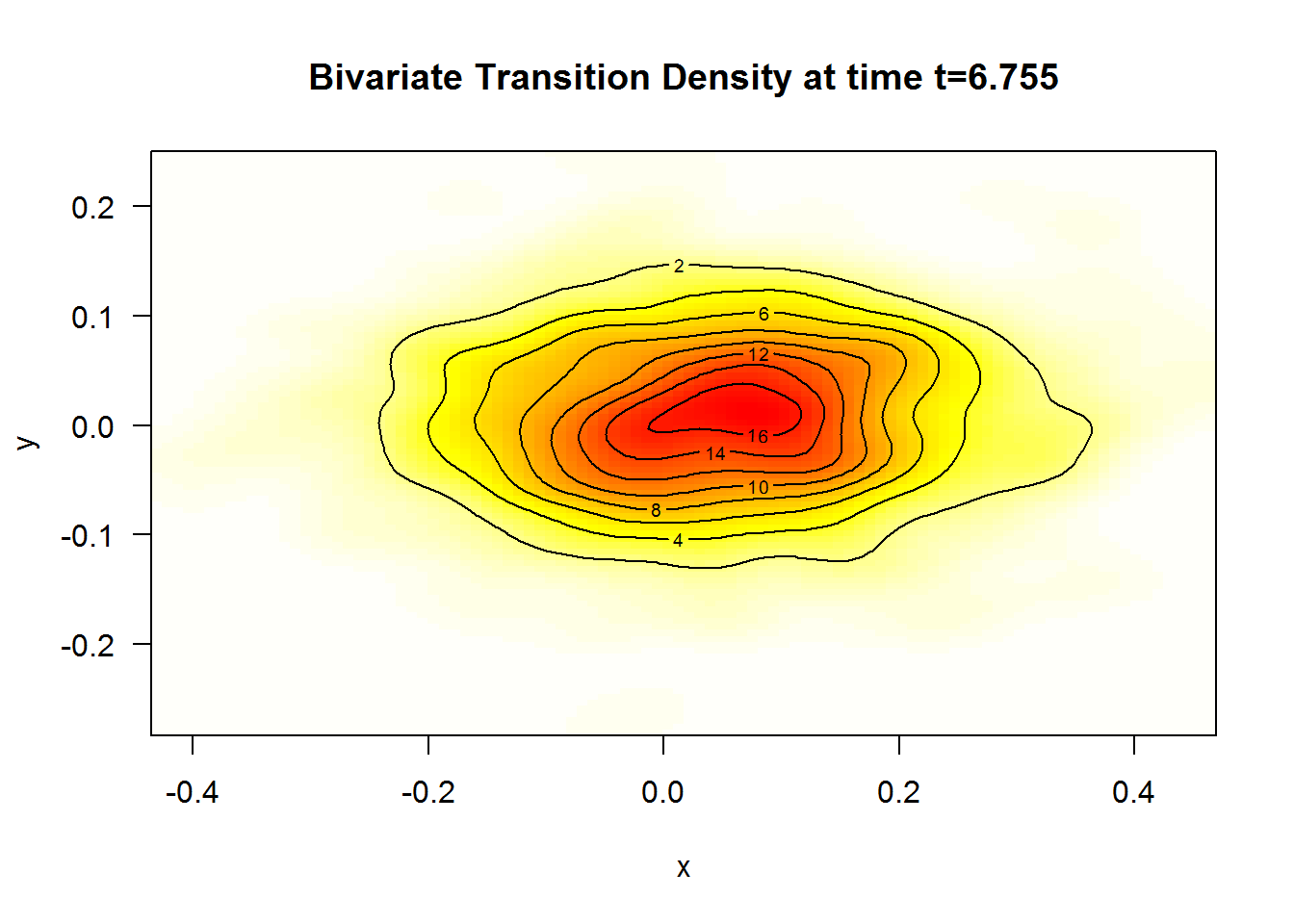A $$3$$D plot of the transition density at $$t=6.75$$ obtained with:

R> plot(denJ,main="Bivariate Transition Density at time t=6.75")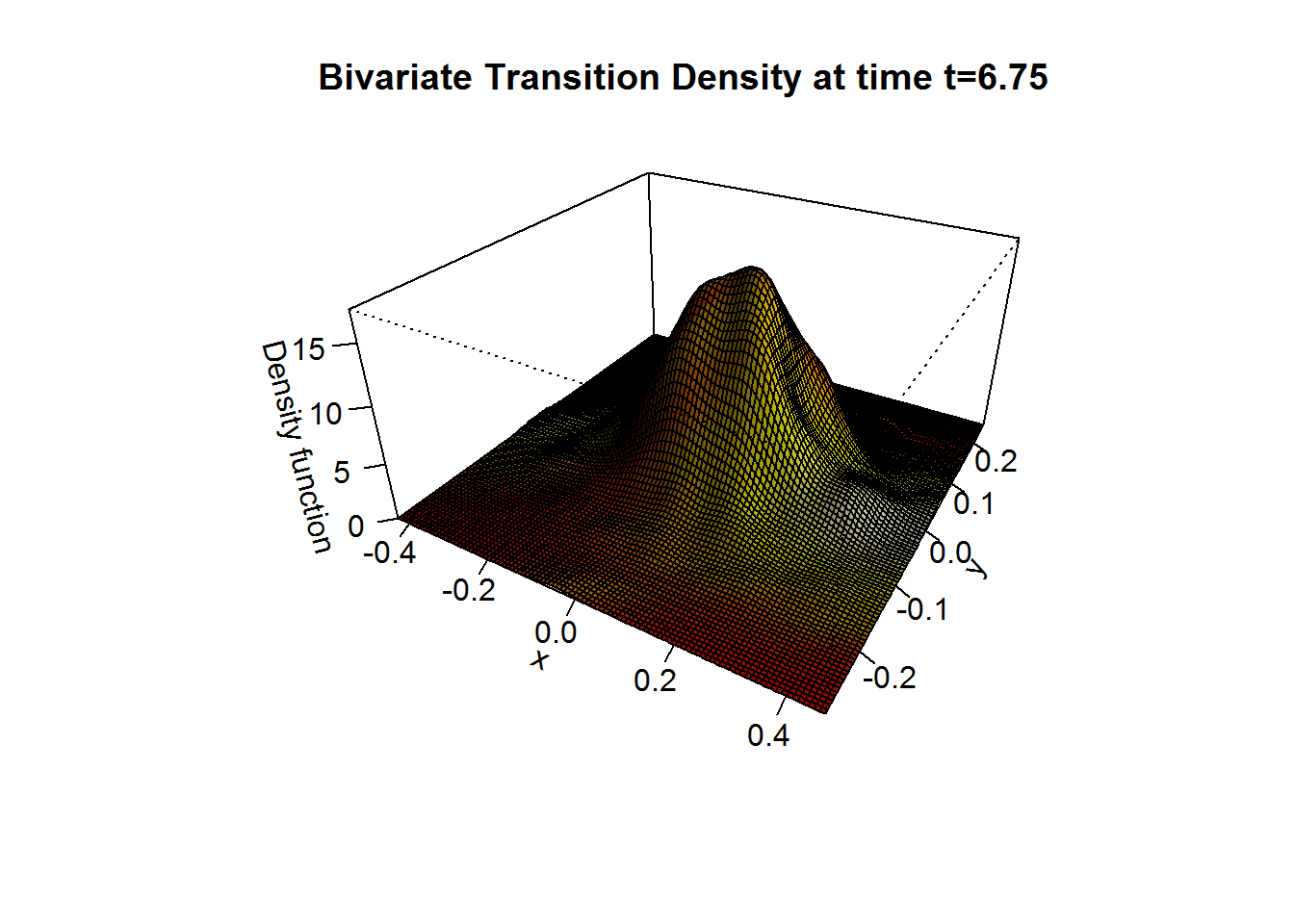We approximate the bivariate transition density over the set transition horizons $$t\in [1,9]$$ with $$\Delta t = 0.005$$ using the code:

R> for (i in seq(1,9,by=0.005)){
+ plot(dsde2d(mod2, at = i,n=100),display="contour",main=paste0('Transition Density \n t = ',i))
+ }

# bridgesde3d()

Assume that we want to describe the following bridges SDE’s (3D) in It? form:

$\begin{equation} \begin{cases} dX_t = -4 (1+X_{t}) Y_{t} dt + 0.2 dW_{1,t},\quad X_{t_{0}}=0 \quad\text{and}\quad X_{T}=0\\ dY_t = 4 (1-Y_{t}) X_{t} dt + 0.2 dW_{2,t},\quad Y_{t_{0}}=-1 \quad\text{and}\quad Y_{T}=-2\\ dZ_t = 4 (1-Z_{t}) Y_{t} dt + 0.2 dW_{3,t},\quad Z_{t_{0}}=0.5 \quad\text{and}\quad Z_{T}=0.5 \end{cases} \end{equation}$

We simulate a flow of $$1000$$ trajectories, with integration step size $$\Delta t = 0.001$$.

R> fx <- expression(-4*(1+x)*y, 4*(1-y)*x, 4*(1-z)*y)
R> gx <- rep(expression(0.2),3)
R> mod3 <- bridgesde3d(x0=c(0,-1,0.5),y=c(0,-2,0.5),drift=fx,diffusion=gx,M=1000)
R> mod3
Itô Bridge Sde 3D:
| dX(t) = -4 * (1 + X(t)) * Y(t) * dt + 0.2 * dW1(t)
| dY(t) = 4 * (1 - Y(t)) * X(t) * dt + 0.2 * dW2(t)
| dZ(t) = 4 * (1 - Z(t)) * Y(t) * dt + 0.2 * dW3(t)
Method:
| Euler scheme with order 0.5
Summary:
| Size of process   | N = 1001.
| Crossing realized | C = 997 among 1000.
| Initial values    | x0 = (0,-1,0.5).
| Ending values     | y  = (0,-2,0.5).
| Time of process   | t in [0,1].
| Discretization    | Dt = 0.001.
R> summary(mod3) ## default: summary at time = (T-t0)/2

Monte-Carlo Statistics for (X(t),Y(t),Z(t)) at time t = 0.5
| Crossing realized 997 among 1000
X        Y        Z
Mean              0.67173  0.50848  0.14427
Variance          0.01301  0.00711  0.02035
Median            0.67589  0.51259  0.14111
Mode              0.67747  0.52734  0.13841
First quartile    0.60427  0.45310  0.05082
Third quartile    0.75209  0.56303  0.23480
Minimum           0.18638  0.23710 -0.35196
Maximum           1.01699  0.74816  0.65446
Skewness         -0.36067 -0.15925  0.07065
Kurtosis          3.59540  3.04480  3.25344
Coef-variation    0.16977  0.16582  0.98880
3th-order moment -0.00053 -0.00010  0.00021
4th-order moment  0.00061  0.00015  0.00135
5th-order moment -0.00008 -0.00001  0.00003
6th-order moment  0.00005  0.00001  0.00015

For plotting (back in time) using the command plot, and plot3D in space the results of the simulation are shown in Figure 12:

R> plot(mod3) ## in time
R> plot3D(mod3,display = "persp",main="3D Bridge SDE's") ## in space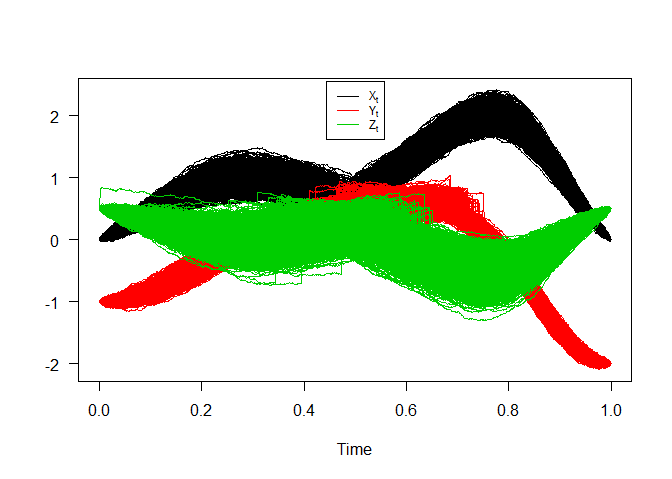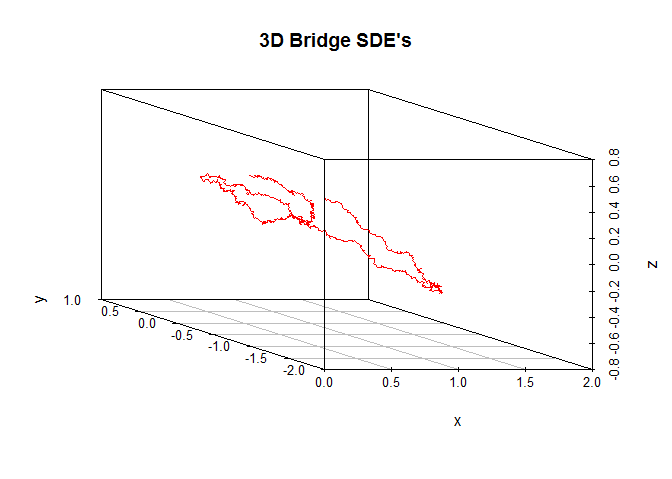Bridge sde 3D

Figure 13, show approximation results for $$m_{1}(t)=\text{E}(X_{t}|X_{0}=0,X_{T}=0)$$, $$m_{2}(t)=\text{E}(Y_{t}|Y_{0}=-1,Y_{T}=-2)$$, $$m_{3}(t)=\text{E}(Z_{t}|Z_{0}=0.5,Z_{T}=0.5)$$ and $$S_{1}(t)=\text{V}(X_{t}|X_{0}=0,X_{T}=0)$$, $$S_{2}(t)=\text{V}(Y_{t}|Y_{0}=-1,Y_{T}=-2)$$, $$S_{3}(t)=\text{V}(Z_{t}|Z_{0}=0.5,Z_{T}=0.5)$$,

R> m1  <- apply(mod3$X,1,mean) R> m2 <- apply(mod3$Y,1,mean)
R> m3  <- apply(mod3$Z,1,mean) R> S1 <- apply(mod3$X,1,var)
R> S2  <- apply(mod3$Y,1,var) R> S3 <- apply(mod3$Z,1,var)
R> out3 <- data.frame(m1,m2,m3,S1,S2,S3)
R> matplot(time(mod3), out3, type = "l", xlab = "time", ylab = "", col=2:7,lwd=2,lty=2:7,las=1)
R> legend("bottom",c(expression(m(t),m(t),m(t),S(t),S(t),S(t))),col=2:7,lty=2:7,lwd=2,bty="n")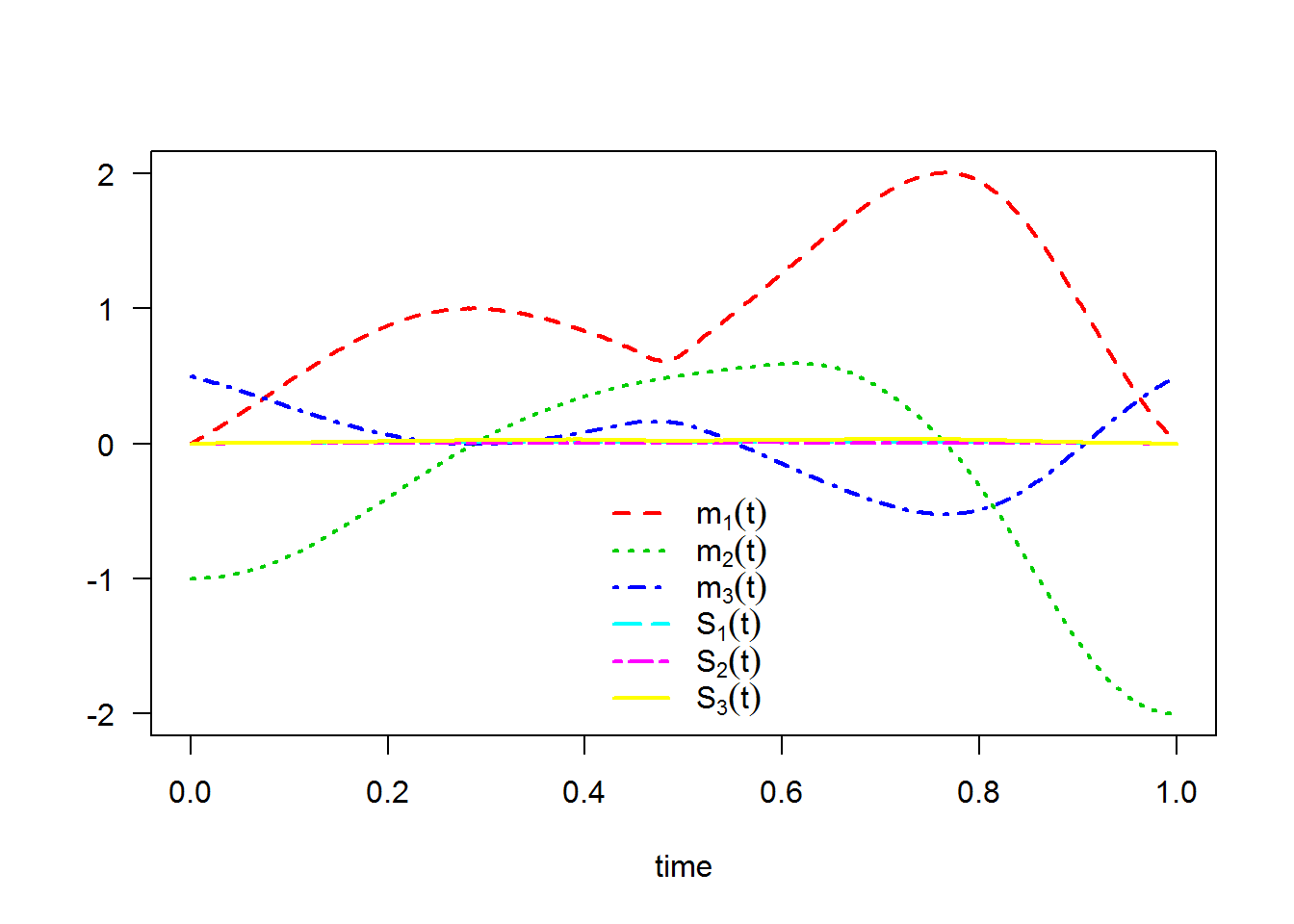The following statistical measures (S3 method) for class bridgesde3d() can be approximated for the $$X_{t}|X_{0}=0,X_{T}=0$$, $$Y_{t}|Y_{0}=-1,Y_{T}=-2$$ and $$Z_{t}|Z_{0}=0.5,Z_{T}=0.5$$ process at any time $$t$$, for example at=0.75:

R> s = 0.75
R> mean(mod3, at = s)
  1.99433  0.11949 -0.51846
R> moment(mod3, at = s , center = TRUE , order = 2) ## variance
 0.0118241 0.0047108 0.0323825
R> Median(mod3, at = s)
  1.99223  0.12046 -0.51403
R> Mode(mod3, at = s)
  1.96615  0.12768 -0.52253
R> quantile(mod3 , at = s)
$x 0% 25% 50% 75% 100% 1.7038 1.9193 1.9922 2.0704 2.2966$y
0%       25%       50%       75%      100%
-0.121572  0.074657  0.120458  0.167431  0.317558

$z 0% 25% 50% 75% 100% -1.14747 -0.63116 -0.51403 -0.39888 0.01747  R> kurtosis(mod3 , at = s)  2.5531 2.8919 3.1096 R> skewness(mod3 , at = s)  -0.0099719 -0.0899127 -0.1462444 R> cv(mod3 , at = s )  0.054551 0.574699 -0.347262 R> min(mod3 , at = s)  1.70383 -0.12157 -1.14747 R> max(mod3 , at = s)  2.29658 0.31756 0.01747 R> moment(mod3 , at = s , center= TRUE , order = 4)  0.000357664 0.000064304 0.003267353 R> moment(mod3 , at = s , center= FALSE , order = 4)  16.10175894 0.00065778 0.12951774 The result summaries of the $$X_{t}|X_{0}=0,X_{T}=0$$, $$Y_{t}|Y_{0}=-1,Y_{T}=-2$$ and $$Z_{t}|Z_{0}=0.5,Z_{T}=0.5$$ process at time $$t=0.75$$: R> summary(mod3, at = 0.75)  Monte-Carlo Statistics for (X(t),Y(t),Z(t)) at time t = 0.75 | Crossing realized 997 among 1000 X Y Z Mean 1.99433 0.11949 -0.51846 Variance 0.01184 0.00472 0.03241 Median 1.99223 0.12046 -0.51403 Mode 1.96615 0.12768 -0.52253 First quartile 1.91934 0.07466 -0.63116 Third quartile 2.07044 0.16743 -0.39888 Minimum 1.70383 -0.12157 -1.14747 Maximum 2.29658 0.31756 0.01747 Skewness -0.00997 -0.08991 -0.14624 Kurtosis 2.55309 2.89188 3.10960 Coef-variation 0.05455 0.57470 -0.34726 3th-order moment -0.00001 -0.00003 -0.00085 4th-order moment 0.00036 0.00006 0.00327 5th-order moment 0.00000 0.00000 -0.00026 6th-order moment 0.00002 0.00000 0.00056 Hence we can just make use of the rsde3d() function to build our random number generator for the triplet $$X_{t},Y_{t},Z_{t}|X_{0}=0,Y_{0}=-1,Z_{0}=0.5,X_{T}=0,Y_{T}=-2,Z_{T}=0.5$$ at time $$t=0.75$$: R> x3 <- rsde3d(object = mod3, at = s) R> head(x3, n = 10)  x y z 1 1.8277 0.123091235 -0.53226 2 1.9193 0.127550704 -0.58236 3 2.0028 -0.000018405 -0.37044 4 2.2095 0.218547309 -0.71229 5 1.9420 0.178317456 -0.62866 6 1.8773 0.136227266 -0.37285 7 2.1056 0.121438357 -0.58440 8 1.9500 0.152582405 -0.51962 9 1.9494 0.144395781 -0.63779 10 1.8688 0.055624684 -0.56250 R> summary(x3)  x y z Min. :1.70 Min. :-0.1216 Min. :-1.1475 1st Qu.:1.92 1st Qu.: 0.0747 1st Qu.:-0.6312 Median :1.99 Median : 0.1205 Median :-0.5140 Mean :1.99 Mean : 0.1195 Mean :-0.5185 3rd Qu.:2.07 3rd Qu.: 0.1674 3rd Qu.:-0.3989 Max. :2.30 Max. : 0.3176 Max. : 0.0175  Display the random number generator for triplet $$X_{t},Y_{t},Z_{t}|X_{0}=0,Y_{0}=-1,Z_{0}=0.5,X_{T}=0,Y_{T}=-2,Z_{T}=0.5$$ at time $$t=0.75$$: , see Figure 14: R> plot(ts.union(mod3$X[,1],mod3$Y[,1],mod3$Z[,1]),col=1:3,lty=3,plot.type="single",type="l",ylab= "",xlab="time",axes=F)
R> points(s,x3$x,pch=19,col=4,cex=0.8) R> points(s,x3$y,pch=19,col=5,cex=0.8)
R> points(s,x3$z,pch=19,col=6,cex=0.8) R> lines(c(s,s),c(-10,x3$x),lty=2,col=7)
R> lines(c(0,s),c(x3$x,x3$x),lty=2,col=4)
R> lines(c(0,s),c(x3$y,x3$y),lty=2,col=5)
R> lines(c(0,s),c(x3$z,x3$z),lty=2,col=6)
R> axis(1, s, bquote(at==.(s)),col=7,col.ticks=7)
R> axis(2, x3$x, bquote(X[t==.(s)]),col=4,col.ticks=4) R> axis(2, x3$y, bquote(Y[t==.(s)]),col=5,col.ticks=5)
R> axis(2, x3$z, bquote(Z[t==.(s)]),col=6,col.ticks=6) R> legend("bottomleft",legend=bquote(c(X[t==.(s)]==.(x3$x),Y[t==.(s)]==.(x3$y),Z[t==.(s)]==.(x3$z))),bty = 'n',cex=0.75)
R> box()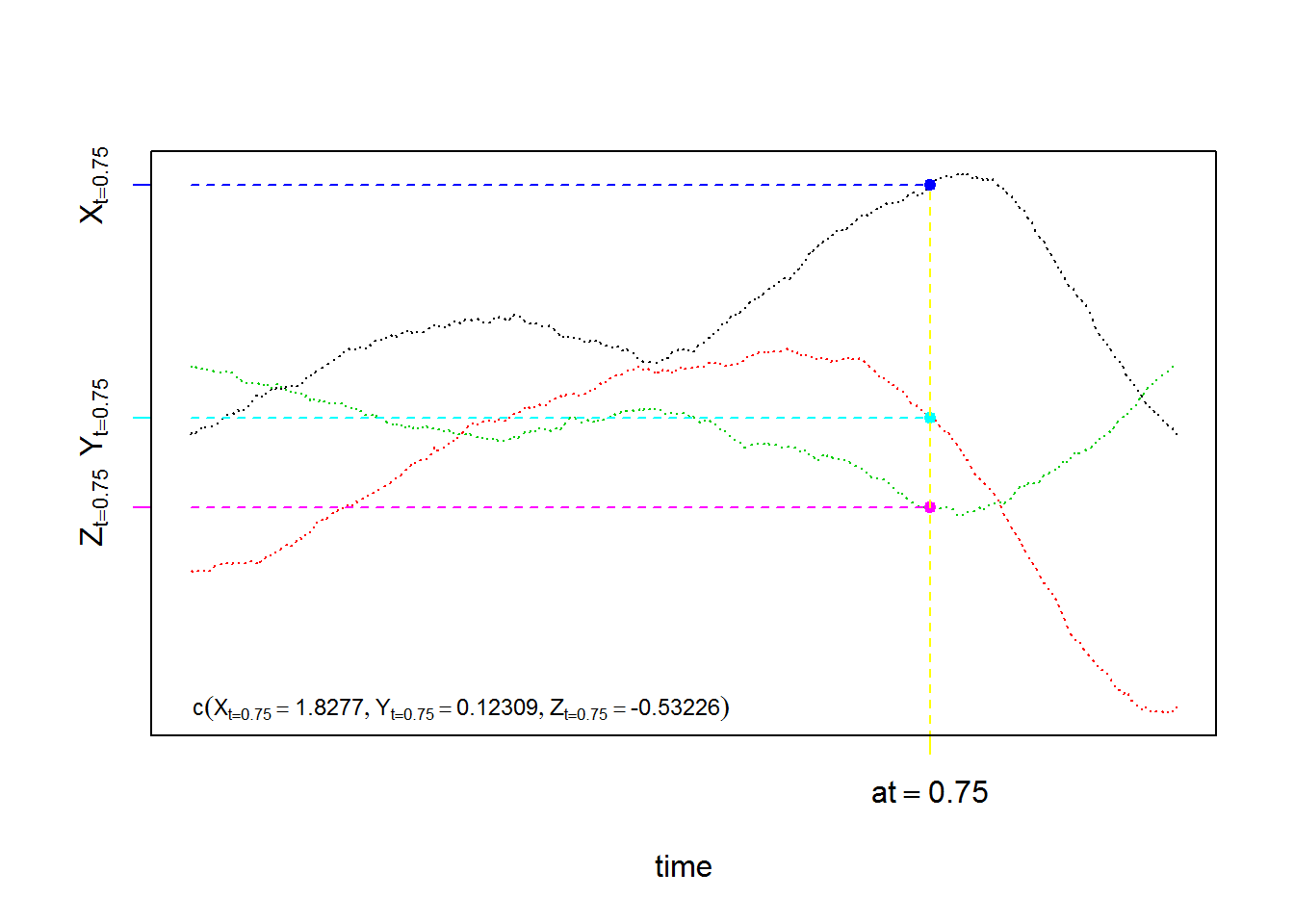For each SDE type and for each numerical scheme, the density of $$X_{t}|X_{0}=0,X_{T}=0$$, $$Y_{t}|Y_{0}=-1,Y_{T}=-2$$ and $$Z_{t}|Z_{0}=0.5,Z_{T}=0.5$$ process at time $$t=0.75$$ are reported using dsde3d() function, see e.g. Figure 15:

R> denM <- dsde3d(mod3,pdf="M",at =s)
R> denM

Marginal density of X(t-t0)|X(t0) = 0, X(T) = 0 at time t = 0.75

Data: x (997 obs.); Bandwidth 'bw' = 0.02461

x               f(x)
Min.   :1.6300   Min.   :0.0002
1st Qu.:1.8151   1st Qu.:0.1381
Median :2.0002   Median :0.9817
Mean   :2.0002   Mean   :1.3493
3rd Qu.:2.1853   3rd Qu.:2.4768
Max.   :2.3704   Max.   :3.4084

Marginal density of Y(t-t0)|Y(t0) = -1, Y(T) = -2 at time t = 0.75

Data: y (997 obs.); Bandwidth 'bw' = 0.01553

y                 f(y)
Min.   :-0.16817   Min.   :0.0003
1st Qu.:-0.03509   1st Qu.:0.0745
Median : 0.09799   Median :0.9516
Mean   : 0.09799   Mean   :1.8767
3rd Qu.: 0.23108   3rd Qu.:3.5991
Max.   : 0.36416   Max.   :5.7643

Marginal density of Z(t-t0)|Z(t0) = 0.5, Z(T) = 0.5 at time t = 0.75

Data: z (997 obs.); Bandwidth 'bw' = 0.03921

z                 f(z)
Min.   :-1.26510   Min.   :0.00015
1st Qu.:-0.91505   1st Qu.:0.05242
Median :-0.56500   Median :0.35151
Mean   :-0.56500   Mean   :0.71348
3rd Qu.:-0.21495   3rd Qu.:1.30398
Max.   : 0.13511   Max.   :2.29072  
R> plot(denM, main="Marginal Density")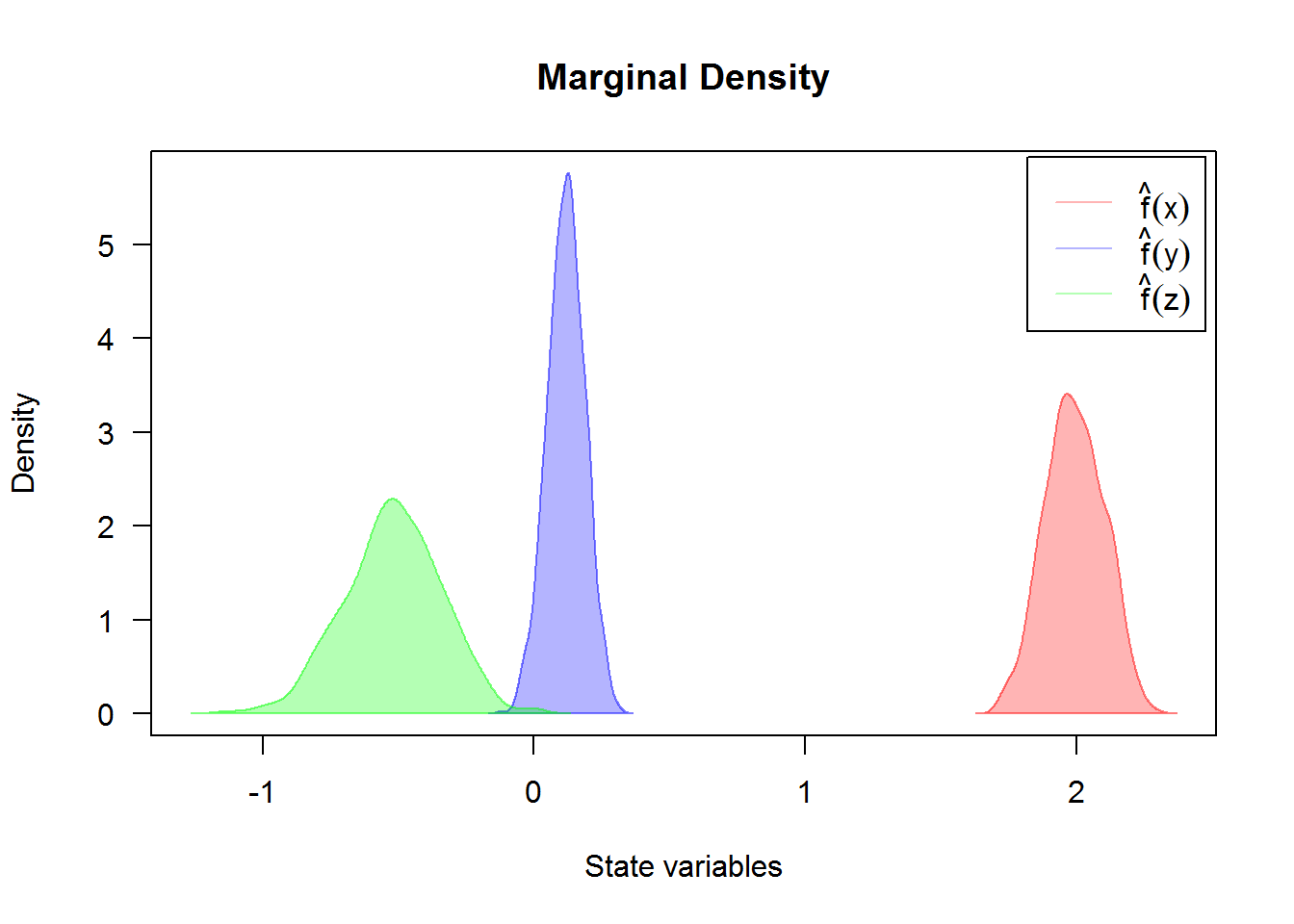For an approximate joint density for triplet $$X_{t},Y_{t},Z_{t}|X_{0}=0,Y_{0}=-1,Z_{0}=0.5,X_{T}=0,Y_{T}=-2,Z_{T}=0.5$$ at time $$t=0.75$$ (for more details, see package sm or ks.)

R> denJ <- dsde3d(mod3,pdf="J",at=0.75)
R> plot(denJ,display="rgl")